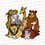# Elementary Techniques used in the IMO (International Mathematical Olympiad) - Invariants

This post follows from Part 5.

Part 6

In this post, we shall explore another method of colouring, more often known as assigning weights. Commonly in such questions, we tend to involve $+1, -1$ a lot since the common technique of taking product and sum gives us lots of additional information. I would advise the reader to take special care as to the motivation behind finding a suitable invariance sum or product as it would help in the exercise in the next post.

(IMO shortlist 2005) There are $n$ markers, each with one side white and the other side black, aligned in a row with their white sides up. At each step, if possible, we choose a marker with the white side up (but not one of the outermost markers), remove it, and reverse the two neighbouring markers. Prove that one can reach a configuration with only two markers left if and only if $3 \nmid n-1$.

Solution: Just playing around with small examples can lead to one trivial observation: the parity of the number of black markers remains unchanged during the game. Hence if only two markers are left, they must have the same colour.

We can see that we can assign "weights". Well, here the best thing to encode would be the number of black markers. Remember that $(-1)^n$ for some $n$ provides lots of information by encoding the parity(when we consider sums), let us define assign to a white marker with $n$ black markers to its left the value $(-1)^n$. We only assign values to the white markers. Denote $\mathbb{S}$ as the sum of all numbers assigned to the white markers.

Consider an arbitrary white marker with both of its neighbours initially white and with $k$ black markers to its left. We perform an operation on that marker and see how it affects $(-1)^n$. Clearly, if we turn both of its neighbouring markers black and remove it, then $\mathbb{S}$ decreases by $-(-1)^k + (-1)^{k-1} + (-1)^{k-1} = 3 (-1)^{k-1}$. Therefore, we have just shown that $\mathbb{S} \pmod 3$ is invariant.

If the game ends with two black markers then $\mathbb{S} \equiv 0 \pmod 3$ and if it ends with two white markers then $\mathbb{S} \equiv 2 \pmod 3$. $\rightarrow 3 \nmid n-1$.

To show that if we start with $n ≥ 5$ markers and $n \equiv 0,2 \pmod 3$ satisfies the conditions. By removing the $3$ leftmost white markers (that do not violate the conditions), we obtain a row of $n-3$ markers. By working backwards, we can reach $2$ or $3$ white markers. We are done with the former and for the latter we can reach $2$ black markers. □

The next post will be tomorrow! :)Note by Anqi Li
7 years, 4 months ago

This discussion board is a place to discuss our Daily Challenges and the math and science related to those challenges. Explanations are more than just a solution — they should explain the steps and thinking strategies that you used to obtain the solution. Comments should further the discussion of math and science.

When posting on Brilliant:

• Use the emojis to react to an explanation, whether you're congratulating a job well done , or just really confused .
• Ask specific questions about the challenge or the steps in somebody's explanation. Well-posed questions can add a lot to the discussion, but posting "I don't understand!" doesn't help anyone.
• Try to contribute something new to the discussion, whether it is an extension, generalization or other idea related to the challenge.

MarkdownAppears as
*italics* or _italics_ italics
**bold** or __bold__ bold
- bulleted- list
• bulleted
• list
1. numbered2. list
1. numbered
2. list
Note: you must add a full line of space before and after lists for them to show up correctly
paragraph 1paragraph 2

paragraph 1

paragraph 2

[example link](https://brilliant.org)example link
> This is a quote
This is a quote
    # I indented these lines
# 4 spaces, and now they show
# up as a code block.

print "hello world"
# I indented these lines
# 4 spaces, and now they show
# up as a code block.

print "hello world"
MathAppears as
Remember to wrap math in $$ ... $$ or $ ... $ to ensure proper formatting.
2 \times 3 $2 \times 3$
2^{34} $2^{34}$
a_{i-1} $a_{i-1}$
\frac{2}{3} $\frac{2}{3}$
\sqrt{2} $\sqrt{2}$
\sum_{i=1}^3 $\sum_{i=1}^3$
\sin \theta $\sin \theta$
\boxed{123} $\boxed{123}$

Sort by:

Nice solution, but I have a couple questions:

Don't you also have to consider removing a white marker with a black on at least one side? Also, at the end how do you remove 3 white markers while leaving the others still white?

- 7 years, 4 months ago

I have the same problem as you about considering the other cases. However, I think I can explain the second part of your question: I initially we have WWWWWW......WWWWWW, ie. n whites. Let us apply the operation to the white second from the left (the first legal white). This leaves us with: BBWWWWW.....WWWWWW, ie. 2 blacks followed by n-3 whites. Now apply the operation to the new first legal white, the white in new position 3. This leaves us with: BWBWWWW...WWWWWWWW, ie. BWB followed by n-5 whites. Finally, apply the operation to the white in position 2 to achieve WWWWWWW...WWWWWWW, ie. n-3 whites.

- 7 years, 4 months ago

Oh yeah I realized that a couple hours after I posted.

- 7 years, 4 months ago

Did you realise why the other cases didn't have to be examined?

- 7 years, 4 months ago

No, I think they do, but I think they work out similarly.

- 7 years, 4 months ago